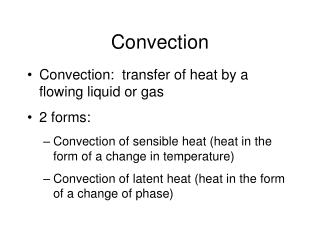DownloadDownload PresentationConvection

# Convection

Télécharger la présentation## Convection

- - - - - - - - - - - - - - - - - - - - - - - - - - - E N D - - - - - - - - - - - - - - - - - - - - - - - - - - -
##### Presentation Transcript

1. Convection • Convection: transfer of heat by a flowing liquid or gas • 2 forms: • Convection of sensible heat (heat in the form of a change in temperature) • Convection of latent heat (heat in the form of a change of phase)

2. Convection of sensible heat • Liquid water has a high volumetric heat capacity CL = 4.17 X 106 J m-3 K-1 (at 20 °C) • Convection of sensible heat can significantly alter the soil thermal regime when liquid water fluxes are relatively large

3. Convection of latent heat • Convective transport of latent heat energy, usually in the form of water vapor. Hv = latent heat of vaporization = 2450 J/g (at 20 °C) • A temperature gradient in moist soil can induce a vapor pressure gradient, which can drive water vapor transport

4. Convection of latent heat

5. Heat conduction • Fourier’s Law: the heat flux is proportional to the temperature gradient • qh = heat flux by conduction (W m-2) •  = thermal conductivity (W m-1 K-1) • T = temperature (K or C) • z = position (m)

6. Transient heat conduction • Heat conservation equation energy in - energy out = change in heat stored • C = Volumetric heat capacity also written crt: mass-based heat capacity, times the wet bulk density (to convert to a volume basis)

7. Transient heat conduction • Combine Fourier’s law: with the heat conservation equation: to describe transient heat conduction:

8. Soil thermal properties • Volumetric heat capacity • Soil thermal conductivity • Soil thermal diffusivity

9. Soil thermal conductivity, k •  is the rate of heat transfer through a unit area of soil under a unit temperature gradient (W m-1 K-1) • Typical values are between 0.1 ≤ ≤ 5 W m-1 K-1 • Varies with Mineralogy Organic matter content Air-filled porosity Water content Temperature Bulk density Spatial arrangement

10. Soil thermal conductivity Volumetric Water Content

11. Volumetric heat capacity, C • C, the volumetric heat capacity, is the energy required to heat a unit volume of soil by one degree. Units: J m-3 K-3 • C varies with • Water content • Bulk density • Mineralogy • Organic matter content • temperature

12. Volumetric heat capacity • C can be estimated by (f is volume fraction) C = fmCm + foCo + fwCw • or byC = wcw + bcs • Typically, 0.8 ≤C≤ 3 MJ m-3 K-1

13. Soil thermal diffusivity, DT • Change in temperature produced in a unit volume by the quantity of heat flowing through the volume in unit time under a unit temperature gradient. • Units: m2 s-1 (same as molecular diffusion) • More useful definition: the ratio of the thermal conductivity to the volumetric heat capacity: DT = /C • Makes the heat flow equation more convenient:

14. Soil thermal diffusivity • Used to characterize the rate of transmission of heat through the soil • Influenced by anything that changes k or C. θ